# Drawing 2D Shapes

Drawing 2D Shapes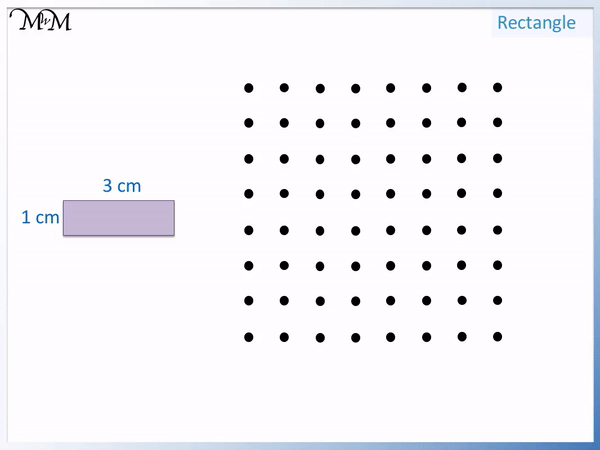• Each dot on this dotted paper is 1 centimetre apart.
• We start by drawing the length of 3 cm by drawing three lines.
• The height of the rectangle is 1 cm so we draw one vertical line.
• Opposite sides on a rectangle are the same length.
• We draw another 3 lines back so that the third line is the same length as the first.
• Rectangles has 4 sides and so we simply connect the end of the third line to the beginning with 1 line.• The only side length that we have labelled is the 2 cm base so we draw this first.
• We draw the height of 2 cm from the middle of this base upwards.
• We then connect this point to the two ends of the base.
• An isosceles triangle is .#### Drawing Triangles and Rectangles on Square Grid Paper Accompanying Activity Sheet# How to Draw 2D Shapes

A 2D shape is a flat shape that can be drawn on paper or displayed on a screen. Unlike 3D shapes, they cannot be picked up or held because they do not exist in real life.

Some common 2D shapes are: Triangles, Rectangles, Squares, Parallelograms, Trapeziums, Kites, Pentagons, Hexagons and Circles.

We will look at drawing some of these shapes using both grid paper and dotted paper.

## Drawing Triangles

Triangles have three sides. In the examples below we will be drawing triangles with bases and heights given to us in centimetres.

We always use a ruler to draw any straight sides.

## Drawing Right-Angled Triangles

A right angled triangle has a base and a height which meet at 90 degrees.

It is easiest to draw

and sides compared to diagonal, sloping sides.

To draw a right-angled triangle, draw the base and the height first and then connect the two ends to form the third side.

In this example we have a right-angled triangle with a base and height both of 2 cm.

We draw the base by drawing 2 horizontal lines between the dots.

We then draw the height by connecting the dots with 2 lines. Each gap is 1 cm wide and so, 2 lines drawn end-to-end form a line that is 2 cm long.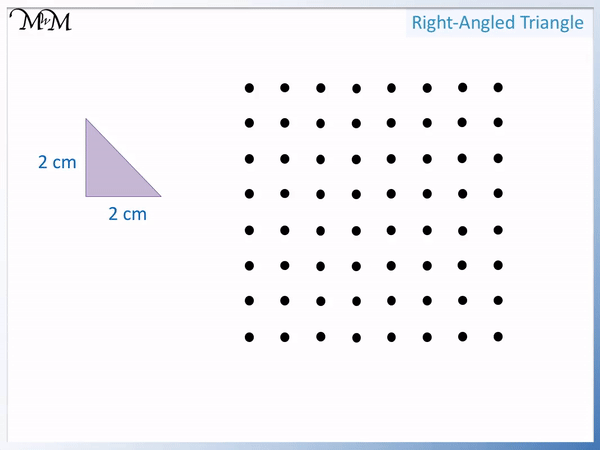We connect the two ends of the base and the height to form the third side.

Because we have measured the other two sides, we do not need to measure this third side, we just connect the two ends with a ruler.

In this example we have a right-angled triangle with a base of 1 cm and a height of 3 cm.We start by drawing the base and height with a ruler.

The base is 1 cm long so we draw one horizontal line.

The height is 3 cm long so we draw 3 lines between the dots.

We now connect the two free ends of these lines to form the third side.

Here is an example of a right-angled triangle with a base of 4 cm and a height of 3 cm.

We draw the base and the height before connecting the two free ends.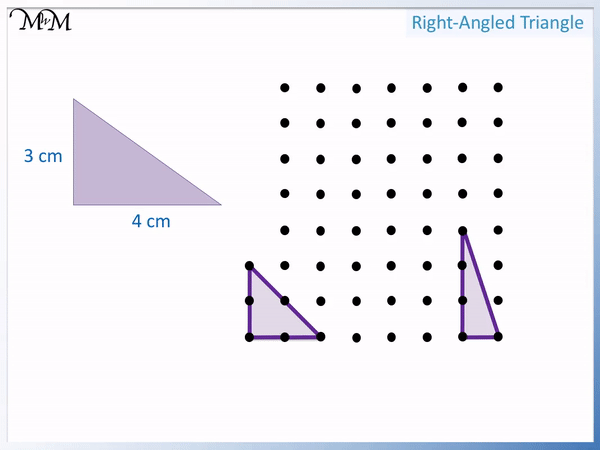Here is an example of drawing a right-angled triangle on grid paper.

It has a base of 5 cm and a height of 1 cm.

Each square on the grid paper is 1 cm apart.Again, we draw the base and height first before joining the two ends.

We draw the base of 5 cm and then the vertical height of 1 cm.

We connect the ends with a ruler.

## Drawing Isosceles Triangles

Isosceles triangles are symmetrical and have two sides the same length.

To draw an isosceles triangle draw the base length first. Measure the height from the middle of the base and mark a point. Connect this point to the two free ends of the base.

In this example we have an isosceles triangle with a base and height of 2 cm.

There is only one side that we know the length of and this is the base. It is 2 cm.

We draw this base first by connecting 2 lines with a ruler.We then count the height upwards from the middle of this base.

The base is 2 cm long, so the middle is 1 cm inwards. We count up 2 cm for the height and mark a point.

We connect this point to each end of the base line.

In this example we have an isosceles triangle with a base of 4 cm and a height of 1 cm.

We know that the base is 4 cm and so we draw 4 lines with a ruler for this base.

We count upwards 1 cm from the middle of this base and mark a point.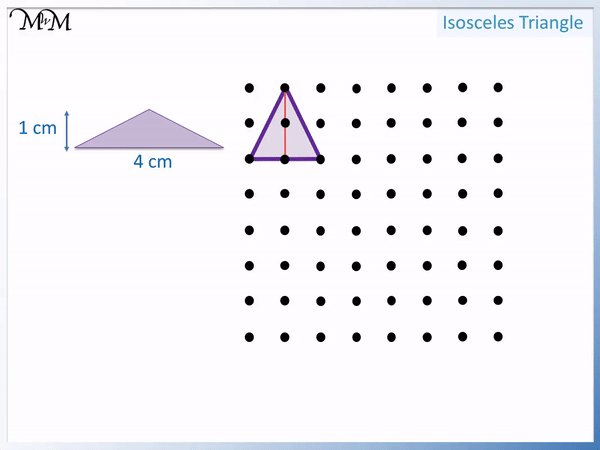We connect this point to each end of the base to complete the triangle.

In this example of an isosceles triangle, we can see that the base of the triangle is vertical. The triangle is on its side.

The only side length we know is the 6 cm side. We draw 6 lines upwards to draw this vertical base side.

The height is 5 cm and so, we count 5 centimetres from the middle of this base line and mark a point.We connect this point to the two ends of the 6 cm line to complete the triangle.

In this example we have an isosceles triangle with a base of 4 cm and a height of 6 cm.

We will draw the triangle on grid paper this time.

The base is 4 cm and we count the height upwards from the middle of this line to mark the top of the triangle.We now connect the top of the triangle to either side of the base line.

## Drawing Rectangles

Rectangles have 4 sides and each side is the same length as the side opposite to it.

Here is an example of a rectangle with a length of 3 cm and a width of 1 cm.

We draw the length first with 3 horizontal lines.

From here we draw the width of 1 cm. We draw one line downwards.Opposite sides on rectangles are the same length.

The base length is the same length as the top length. It is also 3 cm.

Finally we return to the beginning of the rectangle with a 1 cm line upwards.

In the example below we have a rectangle with a width of 2 cm and a length of 4 cm.

We draw 2 horizontal lines for the width and then 4 vertical lines for the length.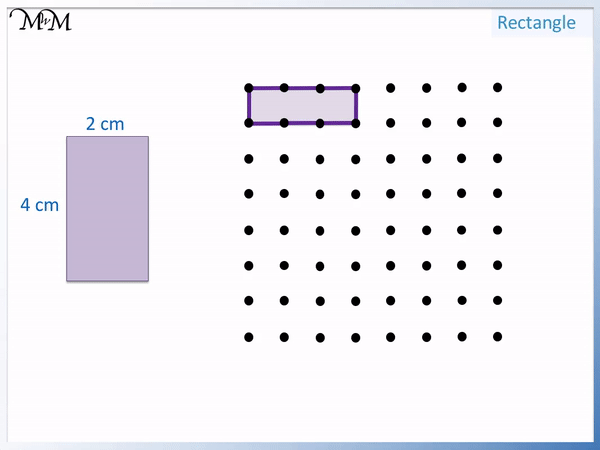The opposite side lengths are then the same length.

Here is an example of drawing a rectangle on grid paper.

We have a rectangle with a base of 5 cm and a height of 3 cm.We count 5 lines horizontally and 3 lines vertically.

We draw the opposite sides to compete the rectangle.

## Drawing Squares

A square is a special kind of rectangle that has all four sides the same length.

Here is an example of a square which has side lengths of 1 cm.

We draw a horizontal line 1 cm long and then a vertical line 1 cm down.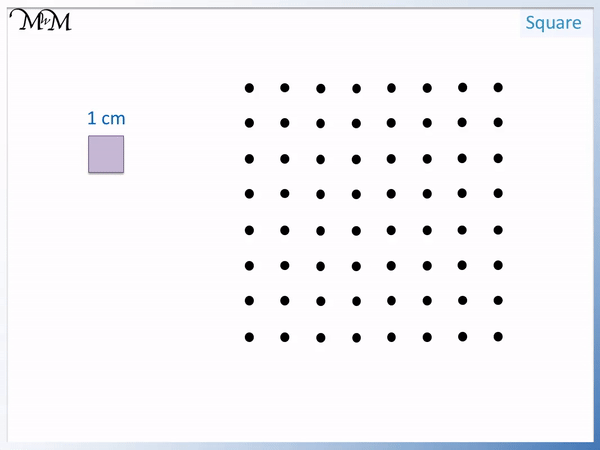Again a square has all of its sides the same length. We go left 1 cm and finally we go up 1 cm.

Here is an example of drawing a square with side lengths of 2 cm.

Again all sides are the same length.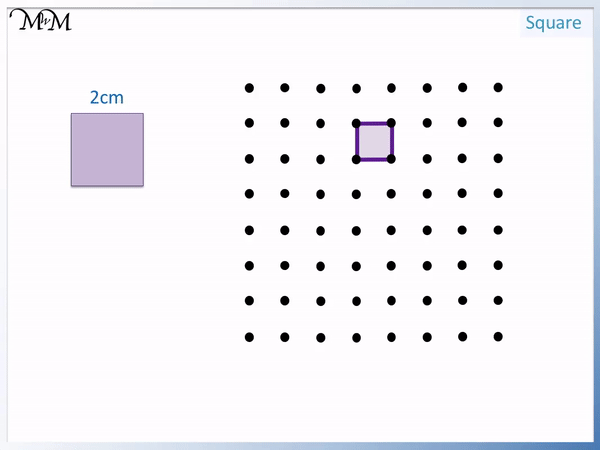Here we have a square with side lengths of 5 cm.

We will draw this square using square grid paper.

We draw 5 lines horizontally and 5 lines vertically.We draw the remaining lines 5 cm long to complete the square.Now try our lesson on Naming 2D Shapes Using Sides and Corners where we learn how to find the names of some common 2D shapes.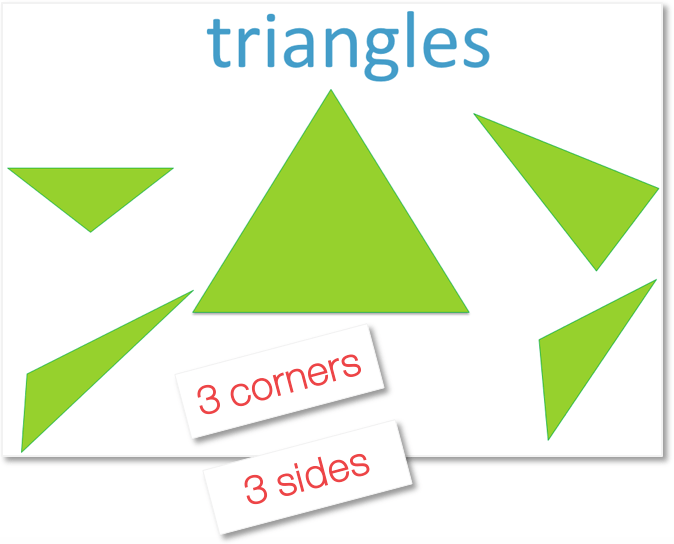error: Content is protected !!